Courses

# Test: The Z-Transform

## 20 Questions MCQ Test GATE ECE (Electronics) 2022 Mock Test Series | Test: The Z-Transform

Description
This mock test of Test: The Z-Transform for Railways helps you for every Railways entrance exam. This contains 20 Multiple Choice Questions for Railways Test: The Z-Transform (mcq) to study with solutions a complete question bank. The solved questions answers in this Test: The Z-Transform quiz give you a good mix of easy questions and tough questions. Railways students definitely take this Test: The Z-Transform exercise for a better result in the exam. You can find other Test: The Z-Transform extra questions, long questions & short questions for Railways on EduRev as well by searching above.
QUESTION: 1

###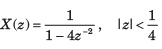​ ​ ​

Solution: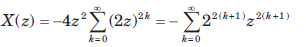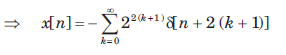QUESTION: 2

### X(z) = ln(1 + z-1), |z| > 0

Solution: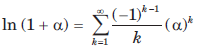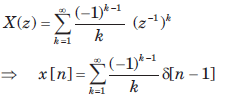QUESTION: 3

### If z-transform is given by X(z) = cos(z-3), |z| > 0 The value of x is

Solution: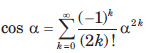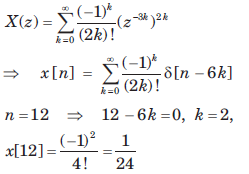QUESTION: 4

X(z) of a system is specified by a pole zero pattern in fig.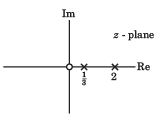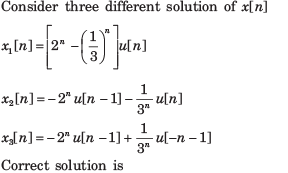Solution:

All gives the same z transform with different ROC. So all are the solution.

QUESTION: 5

Consider three different signal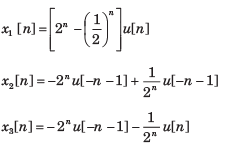fig.shows the three different region. Choose the correct option for the ROC of signal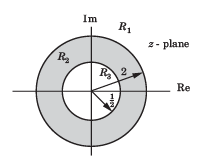R1  , R2 , R3

Solution:

x1[n] is right-sided signal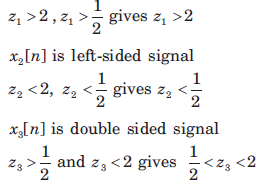QUESTION: 6

Given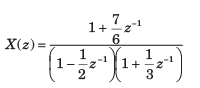For three different ROC consider there different solution of signal x[n] :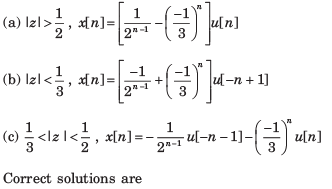Solution: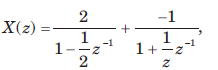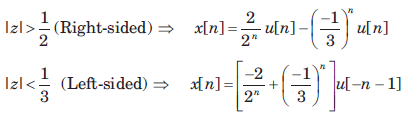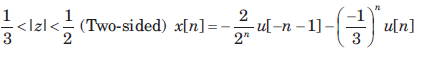QUESTION: 7

X(z) has poles at z =1/2 and z =-1.If x  = 1 x [-1] = 1, and the ROC includes the point z = 34. The time signal x[n] is

Solution:

Since the ROC includes the z = 3/4, ROC is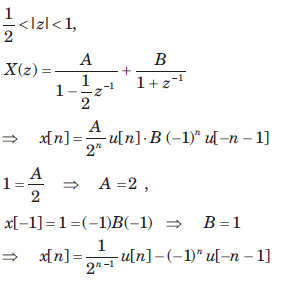QUESTION: 8

x[n] is right-sided, X (z) has a signal pole, and x = 2, x = 1/2. x[n] is

Solution: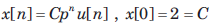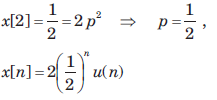QUESTION: 9

The z-transform function of a stable system is given as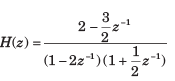The impuse response h[n] is

Solution: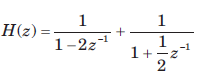h[n] is stable, so ROC includes |z| = 1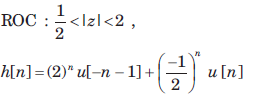QUESTION: 10

Let x[n] = δ[n-2] + δ[n+2]  The unilateral z-transform is

Solution: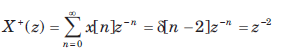QUESTION: 11

The unilateral z-transform of signal x[n] = u[ n + 4 ] is

Solution: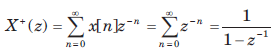QUESTION: 12

The z-transform of a signal x[n] is given by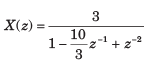If X (z) converges on the unit circle, x[n] is

Solution: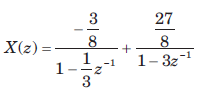Since X(z) converges on |z| = 1. So ROC must include this circle.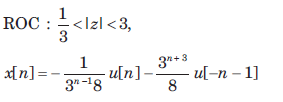QUESTION: 13

The transfer function of a system is given as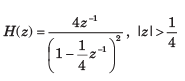The h[n] is

Solution: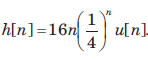So system is both stable and causal. ROC includes z = 1.

QUESTION: 14

The transfer function of a system is given as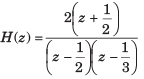Consider the two statements

Statement(i) : System is causal and stable.
Statement(ii) : Inverse system is causal and stable.

The correct option is

Solution: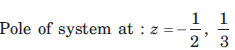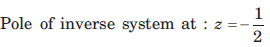For this system and inverse system all poles are inside |z| = 1. So both system are both causal and stable.

QUESTION: 15

The impulse response of a system is given by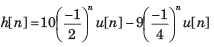For this system two statement are

Statement (i): System is causal and stable

Statement (ii): Inverse system is causal and stable.

The correct option is

Solution:

Pole of this system are inside |z| = 1. So the system is stable and causal.
For the inverse system not all pole are inside |z| = 1. So inverse system is not stable and causal

QUESTION: 16

The system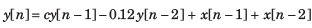is stable if

Solution: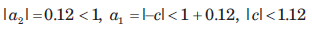QUESTION: 17

Consider the following three systems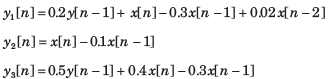The equivalent system are

Solution: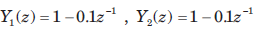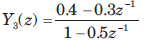So y1 and y2 are equivalent.

QUESTION: 18

The z-transform of a causal system is given as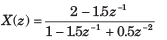The x is

Solution: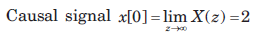QUESTION: 19

The z-transform of a anti causal system is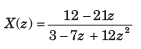The value of x is

Solution: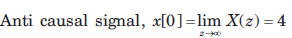QUESTION: 20

Given the z-transforms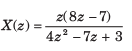The limit of x[ ∞] is

Solution:

The function has poles at z = 1,3/4. Thus final value theorem applies.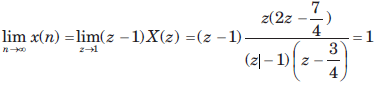• Test